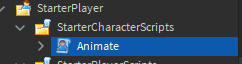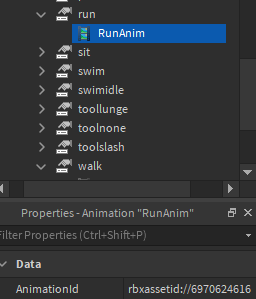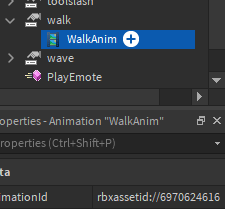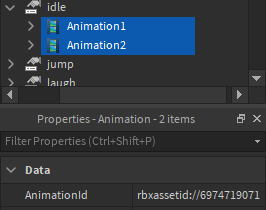# Animation fine for player but looks weird for others

I’m trying to make a walking animation for my game, but I’m having some issues.

For some reason, when players look at each other they see the walking animation but broken.
When you look at yourself walk it is completely fine. This is not only during testing but also when I publish the game.
This is the same for everyone, meaning for them it looks normal but for others it doesn’t.

Player walking and seeing it completely fine.

Player seeing others walk, the animation is weird.

I have tried setting the animation priority to action and have no clue what to do next.

The animation priority is set to movement and I enabled loop.
I have the “Animate” script set within StarterCharacterScriptsThis is the part with all the AnimationId’s inside the "Animate"script

``````local animNames = {
idle = 	{
{ id = "rbxassetid://6974719071", weight = 1 },
{ id = "rbxassetid://6974719071", weight = 1 },
{ id = "rbxassetid://6974719071", weight = 9 }
},
walk = 	{
{ id = "rbxassetid://6970624616", weight = 10 }
},
run = 	{
{ id = "rbxassetid://6970624616", weight = 10 }
},
swim = 	{
{ id = "http://www.roblox.com/asset/?id=507784897", weight = 10 }
},
swimidle = 	{
{ id = "http://www.roblox.com/asset/?id=507785072", weight = 10 }
},
jump = 	{
{ id = "http://www.roblox.com/asset/?id=507765000", weight = 10 }
},
fall = 	{
{ id = "http://www.roblox.com/asset/?id=507767968", weight = 10 }
},
climb = {
{ id = "http://www.roblox.com/asset/?id=507765644", weight = 10 }
},
sit = 	{
{ id = "http://www.roblox.com/asset/?id=2506281703", weight = 10 }
},
toolnone = {
{ id = "http://www.roblox.com/asset/?id=507768375", weight = 10 }
},
toolslash = {
{ id = "http://www.roblox.com/asset/?id=522635514", weight = 10 }
},
toollunge = {
{ id = "http://www.roblox.com/asset/?id=522638767", weight = 10 }
},
wave = {
{ id = "http://www.roblox.com/asset/?id=507770239", weight = 10 }
},
point = {
{ id = "http://www.roblox.com/asset/?id=507770453", weight = 10 }
},
dance = {
{ id = "http://www.roblox.com/asset/?id=507771019", weight = 10 },
{ id = "http://www.roblox.com/asset/?id=507771955", weight = 10 },
{ id = "http://www.roblox.com/asset/?id=507772104", weight = 10 }
},
dance2 = {
{ id = "http://www.roblox.com/asset/?id=507776043", weight = 10 },
{ id = "http://www.roblox.com/asset/?id=507776720", weight = 10 },
{ id = "http://www.roblox.com/asset/?id=507776879", weight = 10 }
},
dance3 = {
{ id = "http://www.roblox.com/asset/?id=507777268", weight = 10 },
{ id = "http://www.roblox.com/asset/?id=507777451", weight = 10 },
{ id = "http://www.roblox.com/asset/?id=507777623", weight = 10 }
},
laugh = {
{ id = "http://www.roblox.com/asset/?id=507770818", weight = 10 }
},
cheer = {
{ id = "http://www.roblox.com/asset/?id=507770677", weight = 10 }
},
}
``````

I have also changed the “Walk”,“Run” and “Idle” tracks within “Animate” to the correct id’s within the script. I have also set the StarterPlayer walk speed to 8.I would kindly appreciate any bit of help possible! Thanks.

1 Like

It was the walkspeed messing with the animation, used this post to help me out after some hours of research.

Its the walk speed. Since it is slow, it is moving awkwardly, and opposed on the other hand, it is not to you.